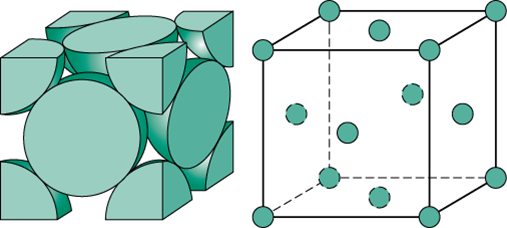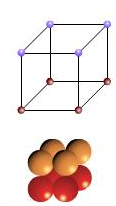#### In a face centred cubic lattice, atom A occupies the corner positions and atom B occupies the face centre positions. If one atom of B is missing from one of the face centred points, the formula of the compound is Option 1)Option 2)Option 3)Option 4)As we learnt in

No. of atoms(z) for face centered unit cell -

Lattice points: at corners and face centers of unit cell.

For face centered cubic (FCC), z=4.

- whereinNo. of atoms(z) for simple cubic unit cell -

Lattice points: at corners

For simple cubic (SC), z=1

- whereinNumber of atom A per unit cellNumber of atom B per unit cellAB5/2 i.e. A2B5

Correct option is 4.

Option 1)This is an incorrect option.

Option 2)This is an incorrect option.

Option 3)This is an incorrect option.

Option 4)This is the correct option.

#### prateek